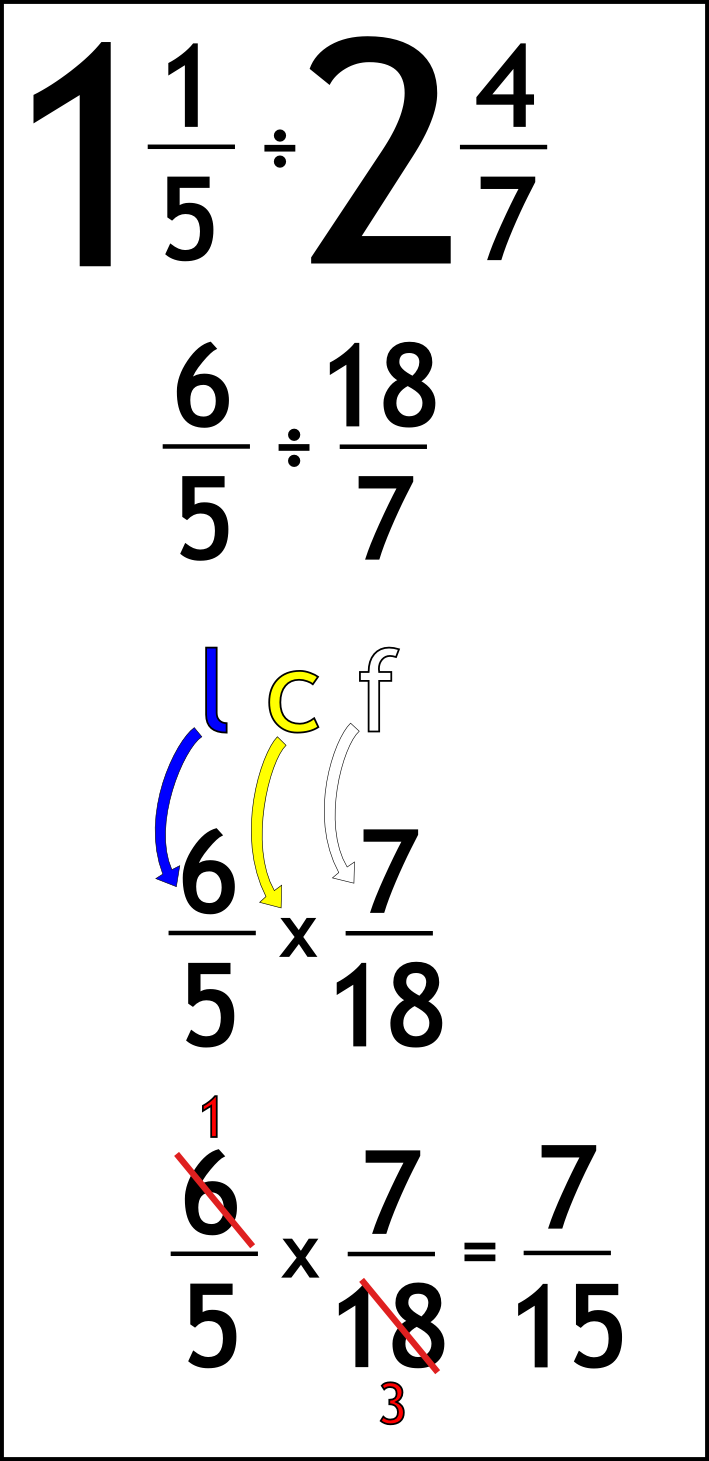The Singing Hedgehog Guide to:
Dividing Fractions 2

As with multiplying with mixed numbers, here too we need fractions first.

Once we just have two fractions we can:

LEAVE IT, CHANGE IT, FLIP IT!

A final example:Write out the question

Turn mixed into top-heavy
1 times 5 is 5 add 1 gives 6
2 times 7 is 14 add 4 gives 18

Do the magic phrase:
leave the first fraction
change the sign to times

flip the second fraction

Now we just have a times question to do
Find common factors (numbers to divide by)

5 and 7 can't simplify further so we leave them

6 and 18 are both divisible by 6 to leave 1 and 3

Multiply across

Job done!

fractions index